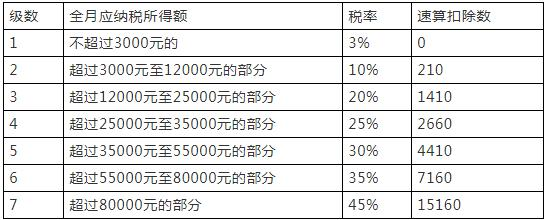C语言编程题目整合

1.数值变换

【问题描述】

【输入形式】

【输出形式】

【输入样例】

356

【输出样例】

653

【样例说明】

【评分标准】

#include <stdio.h>
int main()
{
int a, b, c, d;
scanf("%d",&a);
if(a>=100&&a<1000)
{
b=a/100;
c=a%100/10;
d=a%10;
if(d==0&&c==0)
printf("%d",b);
else if(d==0)
printf("%d%d",c,b);
else
printf("%d%d%d",d,c,b);
}
else
printf("-1");
}

2.sinx计算公式

【问题描述】

【输入形式】

【输出形式】

【样例输入1】

0.5236 4

【样例输出1】

0.50000105

【样例输入2】

0.5236 50

【样例输出2】

0.50000106

【样例说明】

【评分标准】

# include<stdio.h>
# include<math.h>

int main()
{
double p=1.0,f,x;//p是阶乘中的变量，总量即分母是f
double s=0,t=-1;
double i,j,n;
scanf("%lf %lf",&x,&n);
s=x;
f=x;
for(i=3;i<=2*n-1;i=i+2)
{    p=p*i*(i-1);

s=s+t*pow(x,i)/p;
t=-t;
}
printf("%.8lf",s);
}

3.个人所得税

【问题描述】2019年1月1月新修订的个税法正式实施，个人所得税由之前的3500元提高到了5000元，还可以减去6项专项附加扣除，主要有子女教育、继续教育、赡养老人、大病医疗、住房贷款利息和住房租金，扣除三险一金和专项附加，工资大于5000元需要缴纳个人所得税，若小于5000元则不需要缴纳。2019年5000起征点个税表如下：【输入形式】用户在第一行输入月收入和三险一金(单位：元），中间用空格分开，第一个数是月收入，第二个数是三险一金
【输出形式】用户待交的个人所得税（单位：元），保留两位小数
【样例输入】10000 1300
【样例输出】160.00
【样例说明】输入的两个数中，第一个表示月收入，第二个数表示扣除的三险一金;输出待交的个人所得税
【评分标准】完全正确20分。提交源文件名为tax.c

#include <stdio.h>
int main()
{
int t;
double m,l,p,tax;
double rate,late;
scanf("%lf%lf",&l,&p);
m=l-p-5000;

if(m<=0)
{
tax=0;
}
else
{
if(m<=3000)
t=1;
else if(m<=12000)
t=2;
else if(m<=25000)
t=3;
else if(m<=35000)
t=4;
else if(m<=55000)
t=5;
else if(m<=80000)
t=6;
else
t=7;

switch(t)
{
case 1:
rate=0.03,late=0;
break;
case 2:
rate=0.10,late=210;
break;
case 3:
rate=0.20,late=1410;
break;
case 4:
rate=0.25,late=2660;
break;
case 5:
rate=0.30,late=4410;
break;
case 6:
rate=0.35,late=7160;
break;
case 7:
rate=0.45,late=15160;
}
tax=m*rate-late;
}
printf("需要缴纳的个人所得税为:%.2f 元",tax);
return 0;
}

4.字符串复制

【问题描述】

【输入形式】

【输出形式】

【样例输入】

This is a C Program

【样例输出】

Ti saCporm

#include <stdio.h>
#include <string.h>

char *strCopy(char a)
{
static char b;
int j = 0;
for (int i = 0; i < 20; i += 2, j++)
{
b[j]=a[i];
}
return b;
}

int main()
{
char a;
char *p = "";

gets(a);
printf("%s", strCopy(a));
}

5.还是三角形

【问题描述】

【输入形式】

【输出形式】

【样例输入】

3 3 3

【样例输出】

#include <stdio.h>
#include <math.h>
int Max(int a,int b,int c)
{
int t,max;
t=a>b?a:b;
max=t>c?t:c;
return max;
}

int Min(int a,int b,int c)
{
int t,min;
t=a<b?a:b;
min=t<c?t:c;
return min;
}

int Mid(int a,int b,int c)
{
int mid;
mid=a>b?(a<c?a:(b>c?b:c)):(b<c?b:(a>c?a:c));
return mid;
}

int main()
{
int i,n;
int a,b,c,k,x,y,z;
scanf("%d%d%d",&a,&b,&c);
if((a+b>c)&&(abs(a-b)<c))
{//  满足上面条件的三边可以构成三角形
if(a==b&&b==c)
printf("等边三角形\n");
else if((a==b&&c!=a)||(b==c&a!=b)||(a==c&&b!=a))
printf("等腰三角形\n");
else
{
x=Min(a,b,c);
y=Mid(a,b,c);
z=Max(a,b,c);
if(x*x+y*y>z*z)
printf("锐角三角形\n");
else if (a*a+b*b==c*c)
printf("直角三角形\n");
else if(x*x+y*y<z*z)
printf("钝角三角形\n");
}
}
else//不满足构成三角形的条件
{
printf("这三边不能构成三角形\n");
}
}

6.寻找完全数

【问题描述】

【输入形式】

【输出形式】

【样例输入】

32

【样例输出】

28

#include <stdio.h>

int main()
{
long long int m;
long long int n=0,s=1;
long long int i=1,j=2;
long long int c;
scanf("%ld",&m);

for(i=m;i>=1;i--)
{
for(j=2;j<=i;j++)
{
s = i/j;
if(i%j==0)
n = n+s;
}
if(n==i)
{
c = i;
break;
}
n = 0;

}
printf("%ld\n",c);
return 0;
}

7.回文字符串

【问题描述】

【输入形式】

【输出形式】

【样例输入1】

abcdef

【样例输出1】

0

【样例输入2】

abcba

【样例输出2】

5 abcba

【样例输入3】

`aAabccbaABcdcBA

【样例输出3】

8 AabccbaA

#include<stdio.h>
#include<string.h>
#define LEN 100
/*
"最大回文子串是指个字行串中长度最大的回文字符串,其基本算法思想如下：
1、每个回文都有一个"中心"，当回文字符数为奇数时，中间的那个字符就是

2.对任一个字符或者相同的两个连续字符,我们都可以假设它为回文的"中心"，

int Maxhw(char s[]) {
int i,j,k,maxlen;
int start=0;
int slen=strlen(s);
if(slen<1) return 0;
maxlen=0;
for(i=0;i<slen;i++)
{
for(j=0;(i-j>=0)&&(i+j<slen);j++)// 如果以i为中心是奇数的回文子串
{
if(s[i-j]!=s[i+j]) break;
if(j*2+1>maxlen)
{
start=i-j;
maxlen=j*2+1;
}
}
for(j=0;(i-j>=0)&&(i+j+1<slen);j++) // 如果以i为中心是偶数的回文子串
{
if(s[i-j]!=s[i+j+1]) break;
if(j*2+2>maxlen)
{
start=i-j;
maxlen=j*2+2;
}
}
}
for (k=start;k<start+maxlen;k++) //输出最长回文串
printf("%c",s[k]);
printf("\n");
return maxlen; //返回最长回文串的长度
}

int main()
{
char ch[]="aAabccbaABcdcBA";
//char ch[LEN];
//gets(ch);
printf("%d\n",Maxhw(ch));
}

8.三种方法求Fabonacci数列

Fabonacci数列的定义如下：f(n)=f(n−2)+f(n−1) (n≥2)，其中f(0)=0，f(1)=1。

//递归

#include <stdio.h>

int fib(int n)
{
if(n==1||n==2)
return 1;
else
return fib(n-1)+fib(n-2);
}

int main()
{
int n;
scanf("%d",&n);
printf("%d",fib(n));
}
//递推

#include <stdio.h>

int main()
{
int i,n,a=1,b=1;
scanf("%d",&n);
for (i=3;i<=(n+1)/2+1;i++) //递推求相应项的值
{
a=a+b;
b=b+a;
}
if(n%2==1)
printf("%d ",a);
else
printf("%d",b);
}
//数组方法

#include <stdio.h>
int main()
{
int i,n,m;
scanf("%d",&n);
int fib[n];
fib=1;fib=1;
for(i=2;i<n;i++)
{
fib[i]=fib[i-1]+fib[i-2];

}
printf("%d",fib[n-1]);
}

9.相亲数

【问题描述】

2500年前数学大师毕达哥拉斯就发现，220和284两数之间存在着奇妙的联系： 220的因数之和（除了自身之外的因数）为：110+55+44+22+20+11+10+5+4+2+1=284，284的因数之和为：142+71+4+2+1=220。 毕达哥拉斯把这样的数对称为相亲数。输入两个正整数（大于1并且在int的表示范围之内），分别计算它们所有因数之和（除了自身之外的因数），并判断它们是否是一对相亲数。

【输入形式】

【输出形式】

【样例输入1】

220 284

【样例输出1】

220,110+55+44+22+20+11+10+5+4+2+1=284 284,142+71+4+2+1=220 1

【样例输入2】

2560 3282

【样例输出2】

2560,1280+640+512+320+256+160+128+80+64+40+32+20+16+10+8+5+4+2+1=3578 3282,1641+1094+547+6+3+2+1=3294 0

【样例说明】

#include<stdio.h>
int p(int x)
{
int i,n=0;
printf("%d,",x);
for(i=x-1;i>=1;i--)
{
if(x%i==0)
{
printf("%d",i);
n=n+i;
if(i==1)
printf("=");
else
printf("+");
}
}
printf("%d\n",n);
return n;

}
int main()
{
int a,b,v;
scanf("%d %d",&a,&b);
v=p(a);
p(b);
if(v==b)
printf("1");
else
printf("0");

}

10.计算天数

【问题描述】

【输入形式】

【输出形式】

【样例输入】

2003 3 25

【样例输出】

84

【样例说明】

#include<stdio.h>
int Y(int year)
{
int g;
if(year%400==0)
g=1;
else
{
if(year%4==0&&year%100!=0)
g=1;
else
g=0;
}
if(g==1)
{
return 1;
}
else
{
return 2;
}
}

int main()
{
int a,b,c,sum;
int i,k=0,n=0;
scanf("%d %d %d",&a,&b,&c);
if(Y(a)==1&&b>2)
{
for(i=b-1;i>=1;i--)
{
if(i%2==0)
n++;
else
k++;
}
sum=31*k+30*n+c-1;
printf("%d",sum);
}
if(Y(a)==2&&b>2)
{
for(i=b-1;i>=1;i--)
{
if(i%2==0)
n++;
else
k++;
}
sum=31*k+30*n+c-2;
printf("%d",sum);
}
if(b<=2)
{
for(i=b-1;i>=1;i--)
{
if(i%2==0)
n++;
else
k++;
}
sum=31*k+30*n+c;
printf("%d",sum);
}
}

11.求最大公因子

【问题描述】

【输入形式】

【输出形式】

【样例输入】

36 24

【样例输出】

12

【样例说明】

#include<stdio.h>
int f(int x,int y)
{
if(x%y==0)
return y;
else
f(y,x%y);
}
int main()
{
int a,b;
scanf("%d %d",&a,&b);
printf("%d",f(a,b));
}

12.整数合并

【问题描述】

【输入形式】

【输出形式】

【输入样例】

45 12

【输出样例】

5241

#include<stdio.h>
int main()
{
int a,b;
int sum,c,d,e,f;
scanf("%d %d",&a,&b);
c=(a%10)*1000;
a=a/10;
d=(a%10)*10;

e=(b%10)*100;
b=b/10;
f=(b%10);
sum=c+d+e+f;
printf("%d",sum);
}

上一篇C++学习笔记整理-数据的共享与保护

2020-04-23Hexo博客部署到腾讯云服务器(使用宝塔面板)

2020-04-10
目录